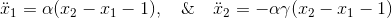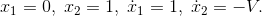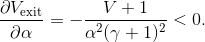# Modelling a tennis impact

By Matthew Haynes

When talking to tennis players and coaches they will readily tell you that increasing the tension of your strings will decrease the power in your strokes. This, at first, seemed counter-intuitive to me. My initial thought process went along the following line. The release of a ball from the racket is similar to the release of a rock from a catapult; the ball gets drawn back into the bed of strings and is then released by tension forces, as in the figure 1. With a catapult, it is apparent that increasing the tension of the attaching ropes increases the speed at which the rock is released. The catapult example has the problem that with a catapult the tension is increased by increasing the length of the elastic, however with a bed of strings the tension is approximately constant throughout the impact. This is due to the large length of the total string and the small change in this length caused by the impact.Figure 1

To analyse this effect, we instead model the string impact process as a ball attached to a spring hitting a plain surface. With this analogy we can include the mass of the racket, its speed and importantly we can compare (atleast qualitatively) the stiffness of the spring to the tension of the strings.  Indeed, we expect a higher tension of the strings to be modelled by higher spring stiffness and this relationship to be approximately linear.Figure 2

Thus to model the contact of the ball in the racket we consider two objects, one modelling the ball with mass m and one modelling the racket with mass M, joined by a spring, with stiffness k, as seen in figure 2. Initially the objects are positioned at the natural length of the spring and are given initial inwards velocities v1, -v2 respectively. The minus comes from the orientation of the velocity and x-axis. Writing this in mathematical terms we must solve,with initial conditions,Where x1, x2 are the non-dimensional positions of the two objects and the constants can be interpreted as follows: α  is the ratio of kinetic energy of the incoming ball to the stored energy in the spring, γ is the ratio of the ball’s mass to that of the racket, and V  is the ratio of the racket’s speed to the ball’s incoming speed. In tennis these typically take values α=2 – 3.7, γ=0.2, V=2. The above system can be solved to find,Therefore the exit speed is given by  where τ is the impact time and can be found by finding the time at which the separation distance returns to the natural length, that is when  in this dimensionless regime. Thus we find,and notice, in particular,Thus, since α has a linear dependence to the string’s tension we find that for all (positive) combinations of α, γ, V, the speed the ball leaves the strings decreases as we increase the tension of the strings.

In summary we see that using a spring to model the impact of a tennis ball on the string predicts that increasing the tension of the strings decreases the speed at which the ball leaves the racket. Which, at least qualitatively, agrees with what coaches tell their players!

Matthew Haynes is a 4th PhD student in MACSI working with Professors Eugene Benilov and Stephen O’Brien. Matt’s work is supported by Science Foundation Ireland Grant Investigator Award No. 12/IA/1683.